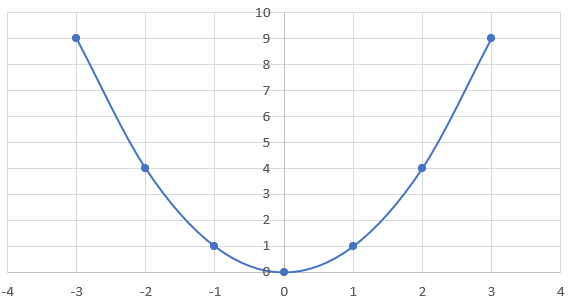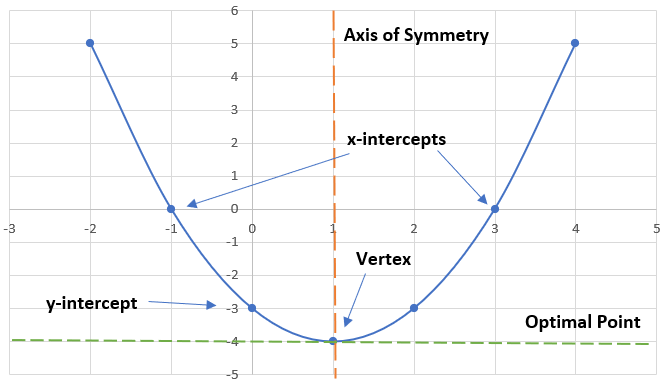Quadratics are Second-Order Polynomials. which take on the form:

$$y = x^2$$

In this form:

• $$x$$ represents the independent variable
• $$y$$ represents the dependent variableA quadratic is referred to as a second-order polynomial since the highest exponent of $$x$$ in a non-zero term is 2.
When a quadratic equation is represented visually, it is referred to as a parabola.

A quadratic function can be expressed algebraically in a few different ways:

• Standard Form: $$y = ax^2 + bx+ c$$
• Vertex Form: $$y = a(x - h)² + k$$
• Factored Form: $$y = a(x - r)(x - s)$$
* We will cover these forms in later lessons!!

## Characteristics of a Parabola

### Direction of Opening

• The sign of $$a$$ (+-) will determine which direction the parabola will open
• If $$a$$ is positive, the parabola will open upwards; if $$a$$ is negative, the parabola will open downwards

### Slope

• Isn't constant unlike a Linear Function
• Pattern can be described as positive, zero, negative.

### Stretch/Compression

• The magnitude of $$|a|$$ will determine whether the parabola will be stretched or compressed
• If $$|a|$$ > 1, the parabola will be stretched, or will appear more narrow
• If $$|a|$$ < 1, the parabola will be compressed, or will appear more wide

### x-intercepts

• Represent the roots, zeroes, or solutions of a quadratic equation
• Points where the parabola crosses the x-axis
• Can be determined algebraically by setting $$y = 0$$ in the quadratic equation

### y-intercept

• The point where the parabola crosses the y-axis
• Can be determined algebraically by setting $$x = 0$$ in the quadratic equation

### Vertex

This point acts as either the minimum or maximum point of a quadratic function.
The characteristics of the vertex depend on the sign of the $$x$$ value in the quadratic equation:

• If $$x$$ > 0 or the parabola opens upward, the vertex will act as a minimum point
• If $$x$$ < 0 or the parabola opens downward, the vertex will act as a maximum point

### Axis of Symmetry

• The vertical line the goes through the vertex
• Determines the x-coordinate of the vertex
• Can be determined algebraically by dividing the sum of the x-intercepts by 2

### Optimal Point

• The hoizontal line that goes through the vertex
• It determines the y-coordinate of the vertex
• Can be determined algebraically by using the Axis of Symmetry as the x-value in the quadratic equationIdentify if the quadratic function $$x^2 + 3$$ has x-intercepts and/or y-intercepts.